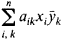# Hermitian Matrix

Also found in: Dictionary, Wikipedia.

## Hermitian matrix

[er′mish·ən ′mā·triks]
(mathematics)
A matrix which equals its conjugate transpose matrix, that is, is self-adjoint.

## Hermitian Matrix

(or self-adjoint matrix), a matrix coincident with its adjoint, that is, a matrix such that aik= āki, where ā is the complex conjugate of the number a. If the elements of a Hermitian matrix are real, then the matrix is symmetric. A Hermitian matrix has real eigenvalues λ1, λ2, …, λn and corresponds to a linear transformation in a complex n-dimensional space that reduces to stretchings by ǀλiǀ in n mutually perpendicular directions and reflections in the planes orthogonal to the directions for which λi < 0. A bilinear formwhose coefficients form a Hermitian matrix, is called a Hermitian form. Any matrix can be written in the form A1 + iA2, where A1 and A2 are Hermitian matrices, and in the form A ∪, where A is a Hermitian matrix and U is a unitary matrix. If A and B are Hermitian matrices, then A B is a Hermitian matrix if and only if A and B commute.

References in periodicals archive ?
Assume A is an n x n singular Hermitian matrix. If A has an (n - 1)st-order positive (negative) definite principal submatrix [A.sub.J], then A is positive (negative) semidefinite.
Caption: Figure 1: Spectrum of a large (N = 1000) Hermitian matrix with a Gaussian distribution ([sigma] = 1).
Since all column and row determinants of a Hermitian matrix over H(R) are equal, in  remark 3.1, put
with [PHI] = [[LAMBDA].sup.-1/2][U.sup.H]K[S.sup.[phi].sub.S(p,v)] U[[LAMBDA].sup.-1/2] being a positive Hermitian matrix. Consequently, the maximization of the SINR consists in finding the maximum eigenvalue of [PHI] and its associated eigenvector u.
Lemma 4:  Let R be a Hermitian matrix with diagonal elements denoted by the vector d and eigenvalues denoted by the vector [lambda].
Voronin, Partial indices of unitary and hermitian matrix functions, Sib.
where [PHI]([omega],[sigma]) is the weight matrix and [[PHI].sup.-1/2] denotes the square root of Hermitian matrix [PHI].
According to quantum mechanics, Hamiltonian operator in matrix form is required to be a Hermitian matrix at least for ensuring that [e.sup.-[iota]xtxH] is a unitary operator.
The inversion lemma of Hermitian matrix  is used to solve [MATHEMATICAL EXPRESSION NOT REPRODUCIBLE IN ASCII].
The eigendecomposition of the positive definite Hermitian matrix [R.sub.x] can be written as

Site: Follow: Share:
Open / Close